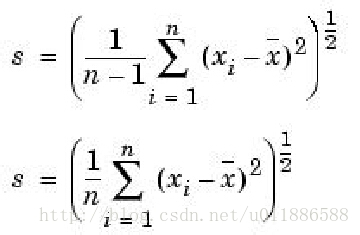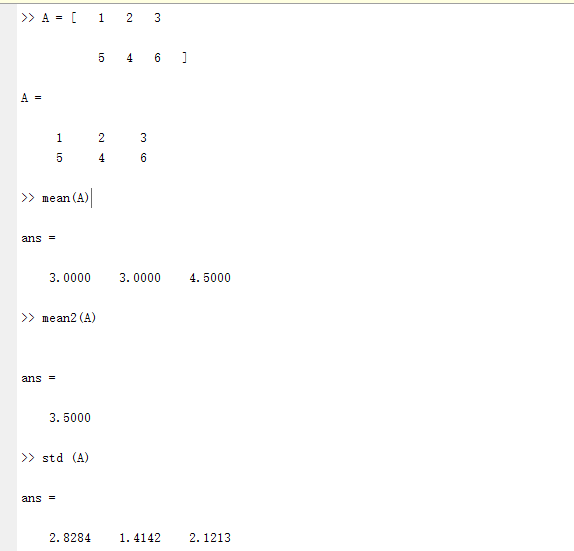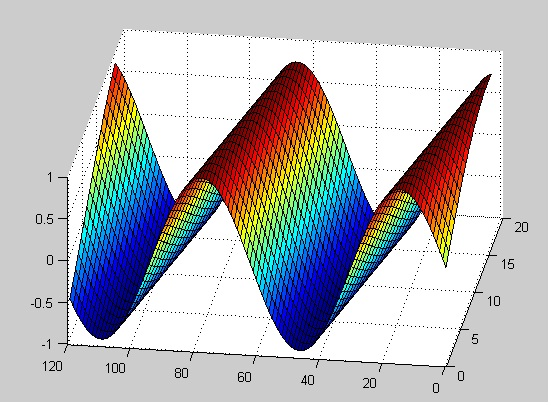• 函数原型：mean(A , dim) dim=1 时，对列求平均数，可省略不写 即 ：mean(A,1) = mean (A) dim=2 时，对行求平均数 2 . mean2() 函数作用 ： 相当于mean( mean( A ) ) 即对整一个矩阵求像素平均值 3 . std() 默认...

1 . mean()

函数作用：求矩阵行或者列的平均数

函数原型：mean(A , dim)

dim=1 时，对列求平均数，可省略不写 即 ：mean(A,1) = mean (A)

dim=2 时，对行求平均数

2 . mean2()

函数作用 ： 相当于mean( mean( A ) )

即对整一个矩阵求像素平均值

3 . std()

默认格式 ： std (A) = std (A,0,1)

函数作用 ： 求标准偏差，即标准差

常见的两种形式：

（1）std( A , a ); std函数的标准形式，

当a=0时，是无偏估计( 概率论有讲过, 累加和除以n-1 )

当a=1时，是有偏估计( 累加和除以n )；（2）std( A , a , b );

增加的b形参代表维数

如果是2维矩阵，b=1是按行分，b=2是按列分

若是3维及以上维数矩阵，b = i,就是增多的一维的维数

验证 ：展开全文• matlab中mean函数是什么意思啊，如何使用在matlab中我知道mean函数是CSS布局HTML小编今天和大家分享均值的，可是mean(x，dim)的dim是什么mean ：平均值或数组的平均值 语法： M = mean(A) M = mean(A,dim) M = ...

matlab中的mean函数是什么意思啊，如何使用

在matlab中我知道mean函数是CSS布局HTML小编今天和大家分享均值的，可是mean(x，dim)中的dim是什么mean ：平均值或数组的平均值 语法： M = mean(A) M = mean(A,dim) M = mean(___,type) 说明： M = mean(A)返回A的大小不等于1的第一个数组维的平均值。 如果A是一个向量，则mean(A)返回元素的平均值。 如果A是一个非空的非向量矩阵。

mean 的意思就是CSS布局HTML小编今天和大家分享平均数。 他的用法可以用于数组 矩阵 语法： mean(X，n) X可以是矩阵或者语法 n是标量 1或者2； 还有其他用法。matlab中 mean(A,2) 什么意思 怎么运算

mean(A) 表示CSS布局HTML小编今天和大家分享矩阵A的均值，默认的是CSS布局HTML小编今天和大家分享各列的均值 如A =[1 2 6; 6 7 2] mean(A) = 3.5000 4.5000 4.0000 mean(A,1) = mean(A) mean(A,2)是矩阵CSS布局HTML小编今天和大家分享各行的均值 如上述A矩阵。

关于MATLAB中的mean函数

matlab mean (A, 3) 是什么意思

对三维以上的矩阵A的第三维数据CSS布局HTML小编今天和大家分享平均 最后结果的第三维大小会变为1 完整的mean函数输入参数有两个 A和dim mean(A,dim) A是待处理的输入矩阵 dim是要CSS布局HTML小编今天和大家分享平均的维 不输入dim时，直接调用 mean(A) 有两种情况 1，当A是数列的时候。

matlab中mean函数的使用

十分不明白mean()中k:12:it的意思，只知道it是sst()中的时间序列，CSS布局HTML小编今天和大家分享解mean(sst(i,k:12:it))的意思是把sst中的第i行，第k列到第it列每隔12个数取一个。

matlab中语句mean(varargin{i}(:))什么意思？

matlab，对于一个三维数组a，mean(a, 1), mean(a, ...mean函数用于计算平均值，在二维数组中，如果把行列看成x和y的话，那么mean(a, 1), mean(a, 2),是分别计算行和列的平均值的，所以，在三维数组中mean(a, 3)使用来计算z方向上的平均值的。

MATLAB 代码：y = mean(x')' 含义？x为矩阵close all; clear; clc; X = [ 1 2 3 4 5 6]; Y = mean(X); % mean 是按照 列CSS布局HTML小编今天和大家分享和 去平均值， Y = mean(X')'; %是按照行CSS布局HTML小编今天和大家分享和取平均值。

展开全文• 如果A是一个向量，mean(A)返回向量各元素的平均值 如果A是一个矩阵，mean(A)将每一列视为一个向量，求每一列的平均值 A=[1,2,3;4,5,6;7,8,9] mean(A) ans = 4 5 6 mean(A,1)和mean(A)的功能一样也是返回...

函数功能：求矩阵或向量的平均值

如果A是一个向量，mean(A)返回向量中各元素的平均值

如果A是一个矩阵，mean(A)将每一列视为一个向量，求每一列的平均值

A=[1,2,3;4,5,6;7,8,9]

mean(A)

ans =

4     5     6

mean(A,1)和mean(A)的功能一样也是返回每一列的均值

mean(A,2)返回每一行的均值

ans =

2
5
8

mean(A(:))返回整个矩阵的均值

ans =

5

mean(A(1,:))返回第一行的均值,其他行以此类推

ans =

2

mean(A(:,1))返回第一列的均值，其他列以此类推

ans =

4

展开全文• matlab中mean函数的用法

万次阅读 2018-09-25 11:08:07
如果A是一个向量，mean(A)返回A各元素的平均值； 如果A是一个矩阵，mean(A)返回的是每一列所有元素的平均值的行向量； M=mean(A,dim) 返回A沿着标量dim指定的维数上的元素的平均值。对于矩阵，mean(A,2)就是包含...

如果A是一个向量，mean(A)返回A中各元素的平均值；
如果A是一个矩阵，mean(A)返回的是每一列所有元素的平均值的行向量；
M=mean(A,dim)
返回A中沿着标量dim指定的维数上的元素的平均值。对于矩阵，mean(A,2)就是包含每一列的平均值的列向量；
A = [1 2 3; 3 3 6; 4 6 8; 4 7 7];
mean(A,1)
ans =
3.0000 4.5000 6.0000
mean(A,2)
ans =
2.0000
4.0000
6.0000
6.0000

本文来自 wonengguwozai 的CSDN 博客 ，全文地址请点击：https://blog.csdn.net/wonengguwozai/article/details/51611270?utm_source=copy

展开全文mean
• MATLAB中的mean函数

千次阅读 2015-09-07 19:13:59
函数功能 求数组的平均数或者均值 使用方法 M = mean(A) 返回沿数组不同维的元素的平均值。 如果A是一个向量，mean(A)返回A元素的平均值。 如果A是一个矩阵，mean(A)将其中的各列视为向量，把矩阵的每列...MATLAB 函数
• MATLAB中mean函数的用法

千次阅读 2018-05-18 15:13:37
mean 平均值或数组的平均值语法：M = mean(A)M = mean(A,dim)M = mean(___,type)说明：M = mean(A)返回A的大小不等于1的第一个数组维的平均值。如果A是一个向量，则mean(A)返回元素的平均值。如果A是一个非空的非...
• 数理统计是信息处理、科学决策的重要理论与方法，其内容丰富，逻辑严谨，实践性强。在统计应用过程matlab是一个非常重要并且方便的软件。matlab中给出了一些统计函数，方便我们更好的进行数据处理，作如下汇总。
• 展开全部mean ：平均值或数组的平均值语法：M = mean(A)M = mean(A,dim)M = mean(___,type)说明：M = mean(A)返回A的大小不等于1的第e69da5e6ba903231313335323631343130323136353331333366306437一个数组维的平均值...
• https://blog.csdn.net/u011886588/article/details/38167859/
• （1） help mean mean Average or mean value.  S = mean(X) is the mean value of the elements in X if X is a vector.   For matrices, S is a row vector containing the mean value of each   colum...
• MATLAB中var函数，mean函数的含义及用法详细解释var函数语句我们先来看var函数，MATLAB命令窗输入help var ，可以得到用到方差函数Syntax有：V = var(X);V = var(X,1);V = var(X,w);V = var(X,w,dim);前两个针对向量...
• 1. mean() 函数定义： numpy.mean(a, axis=None, dtype=None, out=None, keepdims=)[source] Compute the arithmetic mean along the specified axis. Returns the average of the array elements. The average ...
• 1、函数功能 求数组的平均数或者均值2、使用方法 ① M = mean(A) 返回沿数组不同维的元素的平均值。 如果A是一个向量，mean(A)返回A元素的平均值。 如果A是一个矩阵，mean(A)将其中的各列视为向量，把矩阵的每...
• MATLABmean（） 函数的使用

千次阅读 2020-03-17 13:51:49
mean函数可以求矩阵元素的均值 eg: A = 0.3517 0.5497 0.7572 0.8308 0.9172 0.7537 0.5853 0.2858 0.3804 a=mean(A,1) %按列平均 a = 0.5893 0.5843 0.6305 b=mean(A,2) %按行平均 b = 0.5529 0.8339 0.417.....机器学习
• 全栈工程师开发手册 （作者：栾鹏） matlab2c动态链接库下载 matlab库函数大全 ...2、将matlab2c.dll、matlab2c.lib和matlab2c.h放到项目头文件目录下 3、在cpp文件引入下面的代码#include "Matlab2c.h" #pramatlab c++ mean matlab2c
• matlab中求均值的mean()函数的使用

万次阅读 多人点赞 2016-06-08 10:17:05matlab
• Matlabmean()函数的用法

万次阅读 2016-11-21 11:24:13
函数功能 求数组的平均数或者均值 使用方法 M = mean(A) 返回沿数组不同维的元素的平均值。 如果A是一个向量，mean(A)返回A元素的平均值。 如果A是一个矩阵，mean(A)将其中的各列视为向量，把矩阵的每列...matlab
• 函数功能求数组的平均数或者均值使用方法M = mean(A)返回沿数组不同维的元素的平均值。如果A是一个向量，mean(A)返回A元素的平均值。如果A是一个矩阵，mean(A)将的各列视为向量，把矩阵的每列看成一个向量，...
• mean函数 median函数【Matlab】

千次阅读 2019-01-24 10:04:55
8．mean函数 mean函数用于求向量或矩阵的平均值，其调用语法如下。 (1)M=mean(A)：若输入参数A为向量，就返回该向量所有元素的平均值；若为矩阵，则返回每列元素的平均值。 (2)M=mean(A,dim)：返回矩阵A第dim维...
• matlab中求标准差&gt;&gt;A=[1 2 3;4 5 6] &gt;&... std(std(A))结果为0在python可以这样写a = np.array([[1, 2，3], [4, 5, 6]]) ... mean(mean(A))结果为3.5在python可以这...
• [Matlab] mean函数求均值用法

千次阅读 2019-01-20 19:58:15
如果A是一个向量，mean(A)返回A元素的平均值。 如果A是一个矩阵，mean(A)将其中的各列视为向量，把矩阵的每列看成一个向量，返回一个包含每一列所有元素的平均值的行向量。 如果A是一个多元数组，mean(A)将数组...Matlab
• 函数式编程 - MATLAB中的Map函数？我对MATLAB没有地图功能感到有些惊讶，所以我自己一起攻击了一个，因为它是我无法生存的东西。 那里有更好的版本吗？ 有没有一个有点标准的MATLAB函数式编程库我缺少？function ...
• 张智星教授编写的从数据寻找满足某些要求的区间信息的findSegment函数；对数据进行五点三次平滑处理的mean5_3函数；求一组数据的前向预测数据的for_predict函数；求一组数据的前后预测数据的back_predict函数
• MATLAB中stem函数用法

千次阅读 2021-01-17 17:11:56
stem(X,Y)在X的指定点处画出数据序列Y.stem(...,'filled') 以实心的方式画出茎秆.stem(...,'LINESPEC') 按指定的线型画出茎秆及其标记…Matlab中max函数在矩阵函数大小的实例如下:(1)C = max(A)返回一个数组...
• 均值平移跟踪算法函数窗宽的自动选取代码，根据目标大小变化核窗宽，使得当目标出现大小变化时准确跟踪到目标中心
• MATLAB——mean函数

千次阅读 2014-09-18 16:50:57
matlab中mean的用法 函数功能 求数组的平均数或者均值 使用方法 M = mean(A) 返回沿数组不同维的元素的平均值。 如果A是一个向量，mean(A)返回A元素的平均值。 如果A是一个矩阵，mean(A)将...matlab...

matlab中mean2函数matlab 订阅